Courses

# Diode Circuits - 1

## 10 Questions MCQ Test Topicwise Question Bank for GATE Electrical Engineering | Diode Circuits - 1

Description
This mock test of Diode Circuits - 1 for Electrical Engineering (EE) helps you for every Electrical Engineering (EE) entrance exam. This contains 10 Multiple Choice Questions for Electrical Engineering (EE) Diode Circuits - 1 (mcq) to study with solutions a complete question bank. The solved questions answers in this Diode Circuits - 1 quiz give you a good mix of easy questions and tough questions. Electrical Engineering (EE) students definitely take this Diode Circuits - 1 exercise for a better result in the exam. You can find other Diode Circuits - 1 extra questions, long questions & short questions for Electrical Engineering (EE) on EduRev as well by searching above.
QUESTION: 1

### The theoretical maximum efficiency of a bridge rectifier circuit is

Solution:

For a bridge rectifier circuit,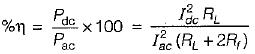For ideal diode,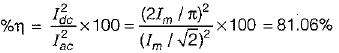and hence maximum efficiency, η = 81.06%.

QUESTION: 2

Solution:
QUESTION: 3

### A full wave rectifier delivers 50 W to a load of 200 Ω. If the ripple factor is 1%, the AC ripple voltage-across the load is

Solution:

DC power delivered to the load is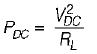∴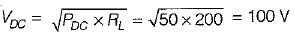The ripple factor,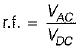or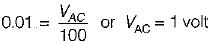Therefore, the AC ripple voltage across the load,
VAC = 1 volt

QUESTION: 4

A half wave rectifier is used to supply 24 V DC to a resistive load of 500 Ω and the diode has a forward resistance of 50 Ω. What is the maximum value of the AC voltage required at the input?

Solution: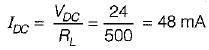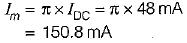Therefore, maximum AC voltage required at the input is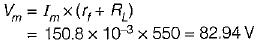QUESTION: 5

What is the value of inductance to be used in the inductor filter connected to a full-wave rectifier operation at 60 Hz to provide a DC output with 4% ripple for a 100 Ω load?

Solution:

The ripple factor for an inductor filter is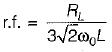∴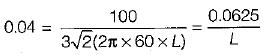or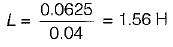QUESTION: 6

Diodes are used to clip voltages in circuits because they act as

Solution:
QUESTION: 7

When a capacitor is connected across the output terminals of a half or full-wave rectifier, the output voltage

Solution:
QUESTION: 8

The equivalent DC output voltage of a full-wave rectifier is ____ the equivalent DC output voltage of a half-wave rectifier.

Solution: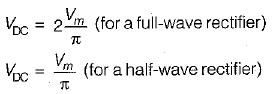QUESTION: 9

Assertion (A): A high value of resistor is connected across the diode in a clamper circuit for better performance.
Reason (R): If this resistor is not present, the circuit will respond to sudden decrease in the magnitude of the input pulse train, but the response to sudden increase in the amplitude will cause improper response.

Solution:
QUESTION: 10

Assertion (A): Bridge arrangement is preferred over centre-tapped transformer arrangement for full-wave rectification.
Reason (R): The bridge arrangement improves the filtering action.

Solution:

No filtering action is provided by bridge arrangement. Hence, reason is a false statement.